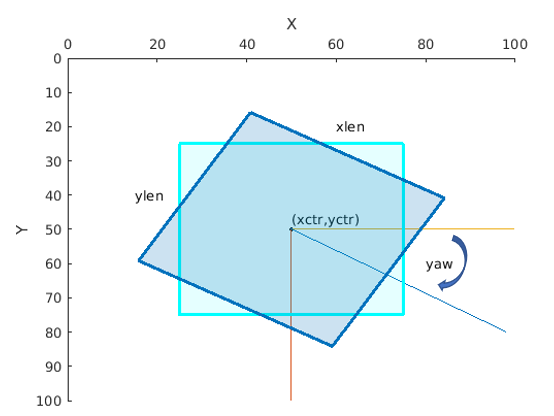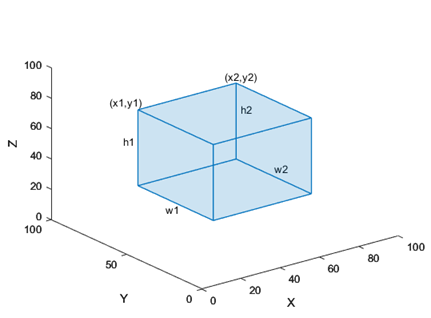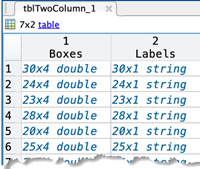# boxLabelDatastore

Datastore for bounding box label data

Since R2019b

## Description

The `boxLabelDatastore` object creates a datastore for bounding box label data. Use this object to read labeled bounding box data for object detection.

To read bounding box label data from a `boxLabelDatastore` object, use the `read` function. This object function returns a cell array with either two or three columns. You can create a datastore that combines the `boxLabelDatastore` object with an `ImageDatastore` object using the `combine` object function. Use the combined datastore to train object detectors using the training functions such as `trainYOLOv2ObjectDetector` and `trainFasterRCNNObjectDetector`. You can access and manage data in the datastore using object functions. To modify the `ReadSize` property, you can use dot notation.

## Creation

### Syntax

``blds = boxLabelDatastore(tbl1,...,tbln)``
``blds = boxLabelDatastore(tbl1,...,tbln,bSet)``

### Description

example

````blds = boxLabelDatastore(tbl1,...,tbln)` creates a `boxLabelDatastore` object from one or more tables containing labeled bounding box data.```
````blds = boxLabelDatastore(tbl1,...,tbln,bSet)` creates a `boxLabelDatastore` object from a `bigimage` data by using the resolution level, block size, and block positions specified by the block locations in `bSet`.```

### Input Arguments

expand all

Labeled bounding box data, specified as a table with one or more columns. Each table corresponds to a set of labels. The bounding boxes can be axis-aligned rectangles, rotated rectangles, or cuboids. The table below describes the format of the bounding boxes.

Bounding BoxDescription
Axis-aligned rectangle

Defined in spatial coordinates as an M-by-4 numeric matrix with rows of the form [x y w h], where:

• M is the number of axis-aligned rectangles.

• x and `y` specify the upper-left corner of the rectangle.

• w specifies the width of the rectangle, which is its length along the x-axis.

• h specifies the height of the rectangle, which is its length along the y-axis.

Rotated rectangle

Defined in spatial coordinates as an M-by-5 numeric matrix with rows of the form [xctr yctr xlen ylen yaw], where:

• M is the number of rotated rectangles.

• xctr and yctr specify the center of the rectangle.

• xlen specifies the width of the rectangle, which is its length along the x-axis before rotation.

• ylen specifies the height of the rectangle, which is its length along the y-axis before rotation.

• yaw specifies the rotation angle in degrees. The rotation is clockwise-positive around the center of the bounding box.Cuboid

Defined in spatial coordinates as an M-by-9 numeric matrix with rows of the form [xctr yctr zctr xlen ylen zlen xrot yrot zrot], where:

• M is the number of cuboids.

• xctr, yctr, and zctr specify the center of the cuboid.

• xlen, ylen, and zlen specify the length of the cuboid along the x-axis, y-axis, and z-axis, respectively, before rotation.

• xrot, yrot, and zrot specify the rotation angles of the cuboid around the x-axis, y-axis, and z-axis, respectively. The xrot, yrot, and zrot rotation angles are in degrees about the cuboid center. Each rotation is clockwise-positive with respect to the positive direction of the associated spatial axis. The function computes rotation matrices assuming `ZYX` order Euler angles [xrot yrot zrot].

The figure shows how these values determine the position of a cuboid.Projected Cuboid

`M`-by-8 vector of the form ```[x1, y1, w1, h1, x2, y2, w2, h2]```, where:

• `M` is the number of labels in the frame.

• `x1, y1` specifies the x,y coordinates for the upper-left location of the front-face of the projected cuboid

• `w1` specifies the width for the front-face of the projected cuboid.

• `h1` specifies the height for the front-face of the projected cuboid.

• `x2, y2` specifies the x,y coordinates for the upper-left location of the back-face of the projected cuboid.

• `w2` specifies the width for the back-face of the projected cuboid.

• `h2` specifies the height for the back-face of the projected cuboid.

The figure shows how these values determine the position of a cuboid.• A table with one or more columns:

All columns contain bounding boxes. Each column must be a cell vector containing M-by-N matrices. M is the number of images and N represents a single object class, such as stopSign, carRear, or carFront.• A table with two columns.

The first column contains bounding boxes. The second column must be a cell vector that contains the label names corresponding to each bounding box. Each element in the cell vector must be an M-by-1 categorical or string vector, where M represents the number of labels.To create a ground truth table, use the Image Labeler or Video Labeler app. To create a table of training data from the generated ground truth, use the `objectDetectorTrainingData` function.

Data Types: `table`

Block locations, specified as a `blockLocationSet` object. You can create this object by using the `balanceBoxLabels` function.

## Properties

expand all

Labeled bounding box data, specified as an N-by-2 cell matrix of N images. The first column must be a cell vector that contains bounding boxes. Each element in the cell contains a vector representing either an axis-aligned rectangle, rotated rectangle, or a cuboid. The second column must be a cell vector that contains the label names corresponding to each bounding box. An M-by-1 categorical vector represents each label name.

Bounding Box Descriptions

Bounding BoxCell VectorFormat
Axis-aligned rectangleM-by-4 for M bounding boxes[x,y,width,height]
Rotated rectangleM-by-5 for M bounding boxes[xcenter,ycenter,width,height,yaw]
CuboidM-by-9 for M bounding boxes[xcenter,ycenter,zcenter,width,height,depth,rx,ry,rz]
Projected cuboidM-by-8 vector for M bounding boxes[x1,y1,w1,h1,x2,y2,w2,h2]

Maximum number of rows of label data to read in each call to the `read` function, specified as a positive integer.

## Object Functions

 `combine` Combine data from multiple datastores `countEachLabel` Count occurrence of pixel or box labels `hasdata` Determine if data is available to read from datastore `numpartitions` Number of partitions for a datastore `partition` Partition a label datastore `preview` Read first row of data in datastore `progress` Percentage of data read from a datastore `read` Read data from a datastore `readall` Read all data in datastore `reset` Reset datastore to initial state `shuffle` Return shuffled version of datastore `subset` Create subset of datastore or FileSet `transform` Transform datastore `isPartitionable` Determine whether datastore is partitionable `isShuffleable` Determine whether datastore is shuffleable

## Examples

collapse all

This example shows how to estimate anchor boxes using a table containing the training data. The first column contains the training images and the remaining columns contain the labeled bounding boxes.

```data = load('vehicleTrainingData.mat'); trainingData = data.vehicleTrainingData;```

Create a `boxLabelDatastore` object using the labeled bounding boxes from the training data.

`blds = boxLabelDatastore(trainingData(:,2:end));`

Estimate the anchor boxes using the `boxLabelDatastore` object.

```numAnchors = 5; anchorBoxes = estimateAnchorBoxes(blds,numAnchors);```

Specify the image size.

`inputImageSize = [128,228,3];`

Specify the number of classes to detect.

`numClasses = 1;`

Use a pretrained ResNet-50 network as a base network for the YOLO v2 network.

`network = resnet50();`

Specify the network layer to use for feature extraction. You can use the `analyzeNetwork` function to see all the layer names in a network.

`featureLayer = 'activation_49_relu';`

Create the YOLO v2 object detection network.

`lgraph = yolov2Layers(inputImageSize,numClasses,anchorBoxes,network, featureLayer)`
```lgraph = LayerGraph with properties: Layers: [182×1 nnet.cnn.layer.Layer] Connections: [197×2 table] InputNames: {'input_1'} OutputNames: {'yolov2OutputLayer'} ```

Visualize the network using the network analyzer.

`analyzeNetwork(lgraph)`

Load a table of vehicle class training data that contains bounding boxes with labels.

```data = load('vehicleTrainingData.mat'); trainingData = data.vehicleTrainingData;```

Add the fullpath to the local vehicle data folder.

```dataDir = fullfile(toolboxdir('vision'),'visiondata'); trainingData.imageFilename = fullfile(dataDir,trainingData.imageFilename);```

Create an `imageDatastore` object using the file names in the table.

`imds = imageDatastore(trainingData.imageFilename);`

Create a `boxLabelDatastore` object using the table with label data.

`blds = boxLabelDatastore(trainingData(:,2:end));`

Combine the `imageDatastore` and `boxLabelDatastore` objects.

`cds = combine(imds,blds);`

Read the data for training. Use the `read` object function to return images, bounding boxes, and labels.

`read(cds)`
```ans=1×3 cell array {128x228x3 uint8} {[126 78 20 16]} {[vehicle]} ```

Load a table of vehicle class training data that contains bounding boxes with labels.

`load('vehicleTrainingData.mat');`

Load a table of stop signs and cars class training data that contains bounding boxes with labels.

`load('stopSignsAndCars.mat');`

Create ground truth tables from the training data.

```vehiclesTbl = vehicleTrainingData(:,2:end); stopSignsTbl = stopSignsAndCars(:,2:end);```

Create a `boxLabelDatastore` object using two tables: one with vehicle label data and the other with the stop signs and cars label data.

`blds = boxLabelDatastore(vehiclesTbl,stopSignsTbl);`

Create an `imageDatastore` object using the file names in the training data tables.

```dataDir = fullfile(toolboxdir('vision'),'visiondata'); vehicleFiles = fullfile(dataDir,vehicleTrainingData.imageFilename); stopSignFiles = fullfile(dataDir,stopSignsAndCars.imageFilename); imds = imageDatastore([vehicleFiles;stopSignFiles]);```

Combine the `imageDatastore` and `boxLabelDatastore` objects.

`cds = combine(imds,blds);`

Read the data for training. Use the `read` object function to return images, bounding boxes, and labels.

`read(cds)`
```ans=1×3 cell array {128x228x3 uint8} {[126 78 20 16]} {[vehicle]} ```

## Version History

Introduced in R2019b

expand all